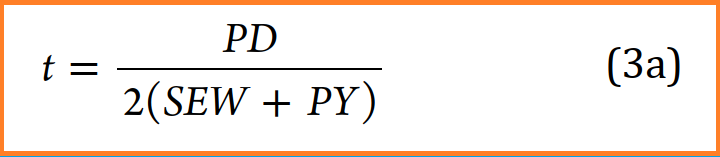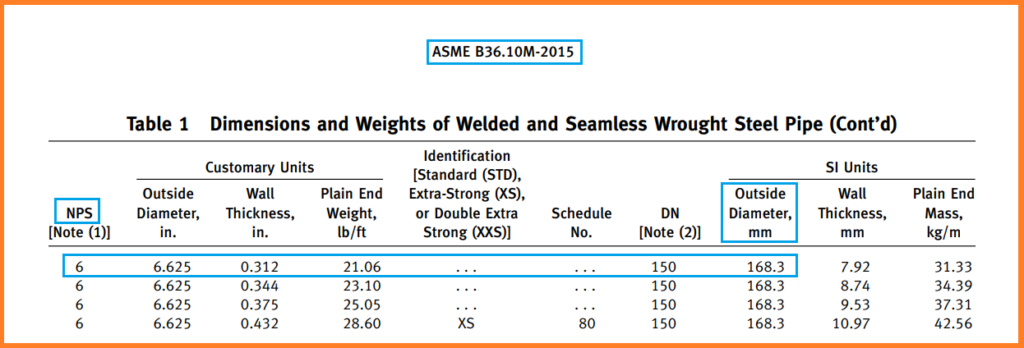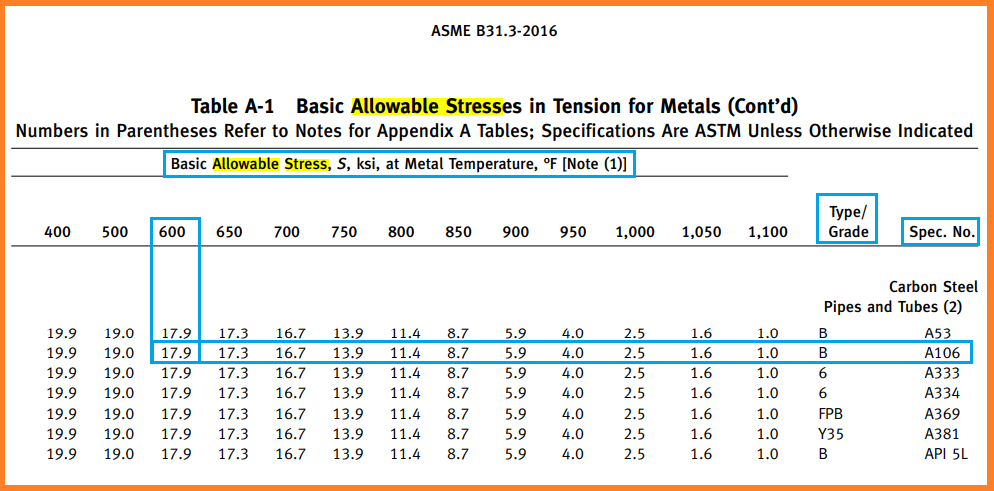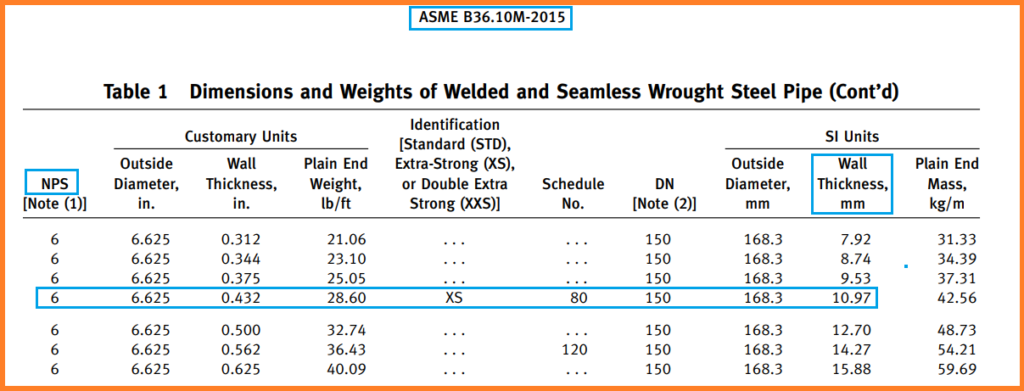# Pipe Thickness Calculation for Internal Pressure

Share with:

Pipe thickness calculation is a very important activity for every piping engineer. As it required to calculate numerous pipe wall thickness calculations for different design conditions.

The process plant piping system deal with the fluids which flow inside the pipe at high-pressure and temperature. So, this high-pressure and temperature fluid causes high circumferential pressure inside the pipe. Hence, it can lead to bursting or damages of the pipe, if the pipe wall thickness is not enough to bare this high-pressure and temperature.

The piping designers need to find out the required pipe thickness as per paragraph “304.1.2 (straight pipe under internal pressure) of ASME B31.3” to bare the inside pressure of the pipe or line.

In this article, we will learn the steps for pipe thickness calculation, uses of extra thickness available in the pipe, and representation of different pipe thicknesses.

## Important Points to know for Pipe thickness Calculation

Before starting the pipe thickness calculation, we (the piping engineers) must know the following points.

• Any plants (mainly process plants) are mainly designed for 20 years or 7200 cycles. (Considering 1 cycle each day; the total number of cycles in 20 years = 20*360=7200 cycles).
• The corrosion allowance of the pipe material is decided by the process department.
• The material engineer decides the pipe material type per fluid nature and design conditions.
• Manufacturing type (seamless or welded) is decided upon the line size and the process requirements.
• You can get line size from the line list or P&ID.
• The pressure and temperature for the line are available in the line list.
• Pressure and temperature may differ from line to line.
• The flowing fluid could be corrosive and toxic in nature per the process requirement.
• Corrosion allowance for wrought steel pipe (CS, LAS & LTCS) is mostly taken 3 mm and zero mm for stainless steel pipes.
• Corrosion allowance is not fixed for all the cases, it may vary from line to line and from project to project.
• Mill tolerance for the seamless pipe is 12.50% and 0.3 mm +/- for the welded (ERW or EFW) pipe.

### Abbreviations

CS – Carbon Steel
LAS – Low Alloy Steel
LTCS – Low-Temperature Carbon Steel
SMLS – Seamless
EFW –
Electric Fusion Weld
ERW –
Electric Resistance Weld

## Input Required for Pipe Thickness Calculation

Following are the required input for pipe wall thickness calculation. We take the same example for thickness calculation-

• MOC (Material of Construction) of the pipe – Carbon Steel (A106 Gr. B)
• NPS (Nominal Pipe Size or Line Size) – 6”
• Manufacturing type of the pipe (SMLS, EFW, ERW) – Seamless (SMLS)
• Design Pressure – 1400 PSIG
• Design Temperature – 600° F
• Mechanical, corrosion, and erosion allowances of the pipe – 3 mm
• Mill Tolerance of the pipe – 12.50%

## Formula for Pipe Wall Thickness Calculation

As per section 304.1.2 of ASME B 31.3, straight pipe thickness calculation under internal pressure is divided into two different conditions –

### (a) If t < D/6Fig. 2: Pipe Thickness Calculation Equation (3a) Under Internal Design Pressure per ASME B 31.3

### (b) If t ≥ D/6 or for P/SE > 0.385

This condition is rarely used. If the above condition ever comes then the calculation of internal design pressure thickness for straight pipe requires special consideration of factors such as the theory of failure,
effects of fatigue, and thermal stress.

Where,

#### P: Internal Design Pressure

for our case study,
P = 1400 PSIG

#### D: Outside Diameter of the pipe

The equation for the pipe wall thickness is based upon the outside diameter of the pipe because the outside diameter of the pipe is constant. Whereas the inside diameter of the pipe varies per the pipe wall thickness.

We can get the outside diameter of pipe from the below standards-

• ASME B36.10M: for wrought steel (CS, LAS & LTCS) Pipes
• ASME B36.19M: for stainless steel pipes

So, let’s find out the outside diameter for our problem – Carbon Steel 6″ NPS.

Hence, need to find the value in ASME B36.10M (refer the below figure).Fig. 3: Pipe Outside Diameter from ASME B 36.10M

So, from fig. 3, D = 168.3 mm

#### S: Allowable Stress value of the Pipe Material (A 106-B) at Design Temperature (500° F)

We need to check allowable value for pipe material (A106 Gr. B) at design temperature (600° F)

Refer Table A-1 (or Table A-1M) of the ASME B31.3, for our case you can refer the below figure (i.e; Fig. 4).Fig. 4: Allowable Stress Value of Pipe Material from Table A-1 of ASME B31.3

For getting the value of the allowable stress. Move-in the horizontal (x) direction for design temperature and move-in vertical (y) direction for pipe material, and the match the both point to get the value (refer to Fig. 4). For our case, it is 17.9 ksi = 17900 psi.

If required, use interpolation to calculate the middle value. For example: at 625° F, the stress value will be between 17.3 and 17.9 ksi, i.e; 17.6 ksi, we can get this value by interpolation.

Important Note: the value of the allowable stress in Table A-1 is given in KSI, So we need to convert the value in PSI for ease of calculation.

#### E: Quality factor for longitudinal weld joints in the pipe

Quality Factors for seamless pipes are 1 for most of the cases and for welded pipes it will differ per the welding method and pipe material.

The Maximum value of quality factors is 1.00

The value of E (Longitudinal Weld Joint Quality Factor) can be found in Table A-1B of the ASME B31.3. The weld joint factor (E) is 1.00 for our problem case as pipe material is A106 seamless (Refer below Fig. 5).

#### W: Weld Joint Strength Reduction Factor

As per para. 302.3.5 of ASME B31.3, The weld joint strength reduction factor (W) is the ratio of the nominal stress to cause the failure of a weld joint to that of the corresponding base material for an increased or elevated temperature condition of the same duration. It only applies at weld joints in longitudinal or spiral welded piping components.

The value of W (weld joint reduction factor) can be found from Table 302.3.5 of ASME B 31.3 (Refer to Fig. 6) and for our problem case, the value of W is 1.

#### Y: Values of Coefficient for t<D/6

The factor “Y” depends upon the temperature. At elevated temperatures, the Y (value of coefficient) increases, and it leads to a decrease in the calculated required pipe wall thickness.

Refer Table 304.1.1 of ASME B31.3 for getting the value of Y, It is Valid for t < D/6. For our case material A106 Gr. B, Y is given 0.4 in the below table (refer to Fig. 7).

## Steps For Pipe Thickness Calculation

Follow the below steps to get the required pipe thickness-

### Step 1:

Put the above found values in the equation (3a) shown in Fig. 2

Therefore,

t = (1400*168.3)/{2(17900*1*1+1400*0.4)} = 235620/36920 = 6.38 mm
Hence, the calculated thickness (t)= 6.38 mm

### Step 2:

Add the corrosion, mechanical and erosion allowance to the calculated thickness

tc = t + c = 6.38 + 3 (for our problem corrosion allowance is 3 mm)
tc = 9.38 mm

### Step 3:

Now, Add the mill tolerance to the tc (thickness after adding corrosion value)

tm = tc + 12.50 % (mill tolerance for seamless pipe is 12.5 %)
=tc/0.875 = 9.38/0.875
tm = 10.72 mm (This is the minimum pipe thickness required to withstands for the given design pressure and temperature).

### Step 4:

Now, check the next nearest thickness available in the ASME B36.10M considering the required thickness (tm). For our case, refer the below fig. 5).Fig. 8: Dimensions and weights of Welded and Seamless Pipe Per ASME B36.10M

Therefore,
from Fig. 8, We can see the Ordering thickness is 10.97 mm or of Schedule 80.

Important Notes:
1.
Ordering thickness for the seamless pipe is always the next greater value available from Schedule to schedule only
2. Whereas for welded pipe any next greater value will be the ordering thickness, for most of the cases it will be next even thickness like 16,18,20,24 mm.
3. Extra thickness available in the pipe can be calculated by ordering thickness minus required thickness = (10.97 – 10.72) = 0.25 mm.

## Uses of the Extra Thickness

Following are the uses of extra thickness available in the pipe-

### 1. For calculating the Life of the pipe after 20 years or 7200 cycles.

We can get the extra life of the pipe with the help of extra thickness available in the pipe.

Extra life of the pipe = extra thickness/corrosion per year = 0.25/0.15 = 1.66 years.

### 2. To calculate the maximum pressure holding capacity of the pipe.

MAWP (Maximum Allowable working pressure) can be calculated using the reverse calculation from the equation (3a) fig. 2

P = [2(T-C)EW/{D-2(T-C)Y}]*S = [2(6.59)*1*1/{168.3 – 2*(6.59)0.4}*17900
where, T-C will be ordering thickness minus mill tolerance (12.5%) minus corrosion allowance (3 mm)

T-C = (10.97*.875)-3 = 6.59 mm

So,
P = (13.18/163.02)*17900 = 1447 PSIG (This will always be higher than the design pressure)

### 3. To check, if the extra thickness is sufficient to take care of thinning if the same pipe is used for manufacture bend.

To get the required thickness for manufacturing bend see the below formula-

Thickness required for bend = Required pipe thickness/0.9 = 10.72/0.9 = 11.91 mm

Here, we can see the thickness required for the bend is greater than the ordering thickness (11.91>10.97). It means we can not make the bend using the same pipe of thickness 10.97 schedules 80. We need to purchase a higher thickness pipe for the above design conditions for manufacturing bend.

## Representation of the Different Thicknesses Available in the Pipe

Where,

t = Calculated thickness = 6.38 mm
ET = Extra thickness = 0.25 mm
CA = Corrosion, mechanical, and erosion allowance = 3 mm
MT = Mill Tolerance = 1.34 mm

I hope You have got the overview of the pipe wall thickness calculation, You can practice for other cases following the mentioned steps for better understanding.

Reference
ASME B31.3

Share with:I'm a graduate in mechanical engineering, and a piping professional. I'm exploring this industry and having a great experience. In the process felt to share my knowledge with the other Piping Engineers and as a result, I am here with you.
View all posts by Rehan Ahmad Khan →

## 9 thoughts on “Pipe Thickness Calculation for Internal Pressure”

1.Myo hlaing says:

Send me hard copy.

2.Masood Eslami Masroor says:

Hi,
could you give me a example based on 302.2.4 of ASME B31.3 (for temporary condition wall thickness calculation)?

3.Simon says:

Keep this going please, great job!

4.Sandeep Gujaran says:

Thanks for this blog. Helped me a lot to calculate pipe thickness for Pharma Project. Can you share Allowable Stress Value of Pipe Material table for Stainless steel SS316L?
Keep doing good Job. i love to read your posts

1.Rehan Ahmad Khan says:

Thanks, Sandeep
Please refer the Table A-1 of ASME B31.3 to get the allowable stress value.

1.Sandeep Gujaran says:

Thank You Rehan. Found it.

5.Sandeep Gujaran says:

Hi Rehan,

Do you know equivalent European Standard to ASME B31.3?

1.Rehan Ahmad Khan says:

Hello Sandeep,

It is EN13480

1.Sandeep Gujaran says:

thank you Rehan,